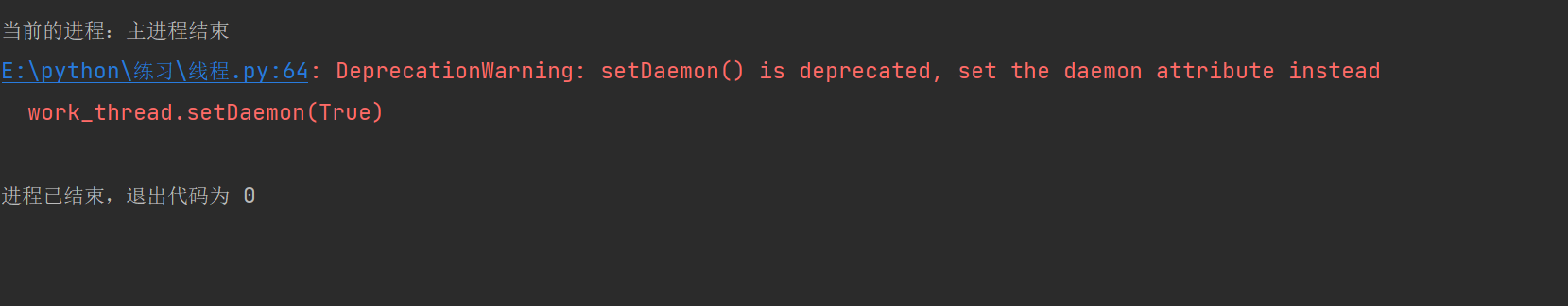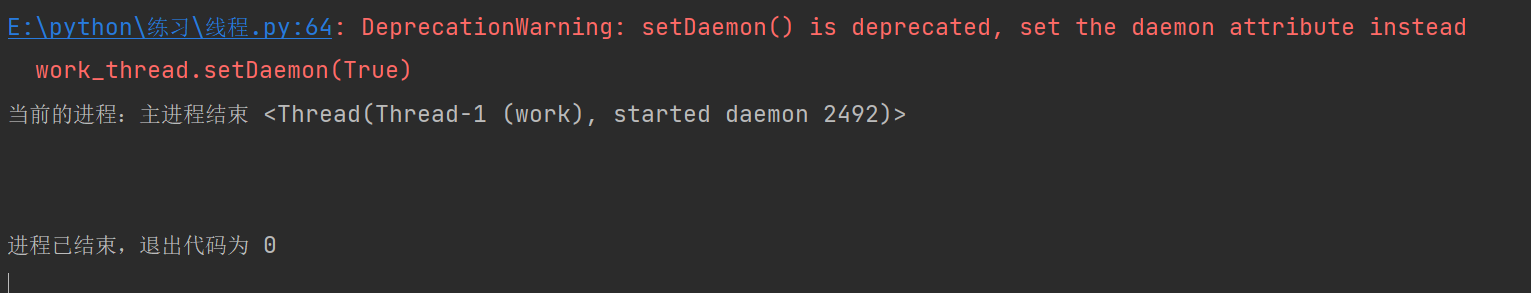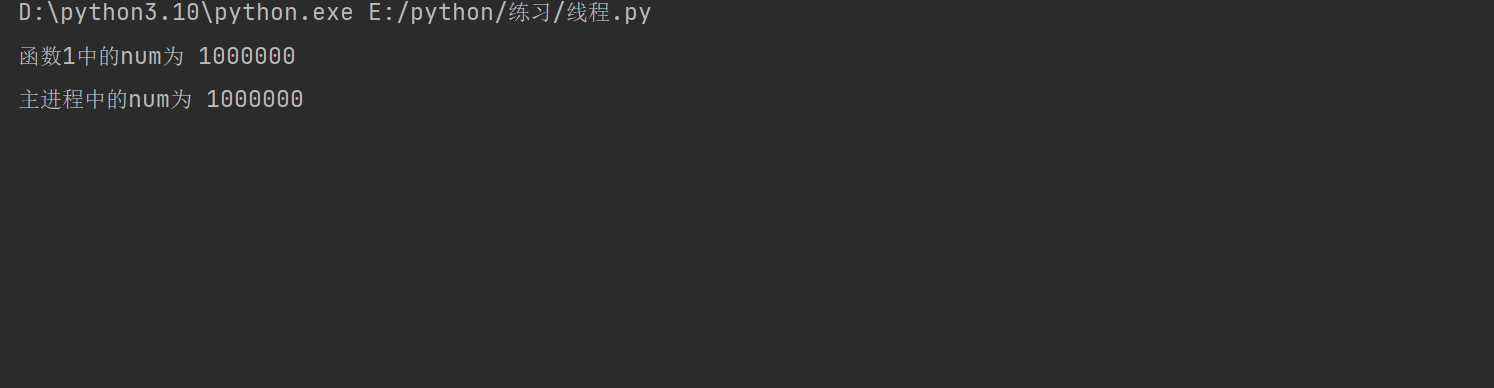### python多任务编程（进程与线程）

Process()

start()

join()

terminate()

current_process()

start()

join()

Lock()

# 在python中多进程的使用

## 模块中的常用命令

### Process()

• group=None,.
• 指定进程组，一般情况下默认即可
• target=None,
• 表示执行的目标任务名(函数、方法名)
• name=None,进程名字
• 表示给这个进程取一个名字，默认为Process-1，依次递增
• args=(),
• 以元组方式给执行任务传参，如果需要为目标函数或者方法传参时，注意一定要是元组，所以当只有一个参数时，需要加一个逗号
• kwargs={}
• 以字典方式给执行任务传参，如果需要为目标函数或者方法传参时，注意一定要是字典
• daemon=None
• 表示该子线程对主线程进行守护操作，使用时daemo的值输入为True，表示守护主进程

## 例子：

### 创建子进程：

``import multiprocessing  def work1():    print('函数1正在工作中')    print('当前进程名为：', multiprocessing.current_process())  # 获取进程名  def work2():    print('函数2正在工作中')    print('当前进程名为：',multiprocessing.current_process())  # 获取进程名 if __name__ == '__main__':    work1_process = multiprocessing.Process(target=work1)    #创建子进程    work2_process = multiprocessing.Process(target=work2,name='进程2')    #创建子进程    work1_process.start()    #运行子进程    work2_process.start()    #运行子进程'''函数1正在工作中当前进程名为： <Process name='Process-1' parent=7648 started>函数2正在工作中当前进程名为： <Process name='进程2' parent=7648 started>'''``

### 对进程的目标传参

``import multiprocessingdef work1(a):   #位置传参    for i in range(a):        print('函数1正在工作中') def work2(sum=None):    #关键字传参    for i in range(sum):        print('函数2正在工作中') if __name__ == '__main__':    work1_process=multiprocessing.Process(target=work1,args=(3,))   #位置传参    work2_process=multiprocessing.Process(target=work2,kwargs={'sum':3})    #关键字传参    work1_process.start()    work2_process.start()'''函数1正在工作中函数1正在工作中函数1正在工作中函数2正在工作中函数2正在工作中函数2正在工作中'''``

## 注意事项

### 进程之间不共享全局变量

``import multiprocessinglist1=[]    #全局变量 def work1():    for i in range(10):        list1.append(i)    print('函数1中的列表为:',list1) def work2():    for i in range(0,20,2):        list1.append(i)    print('函数2中的列表为:',list1) if __name__ == '__main__':    #主进程    work1_process=multiprocessing.Process(target=work1)    work2_process=multiprocessing.Process(target=work2)    work1_process.start()    work2_process.start()    print('全局中的列表为:', list1)'''全局中的列表为: []函数1中的列表为: [0, 1, 2, 3, 4, 5, 6, 7, 8, 9]函数2中的列表为: [0, 2, 4, 6, 8, 10, 12, 14, 16, 18]'''``

### 守护主进程

• deamo = True

• 格式是子进程名.deamo = True。必须放在该子进程开始前。也可以在创建时输入该参数

``import multiprocessingdef work1():    for i in range(3):        print('函数1:',i) def work2():    for i in range(3):        print('函数2:',i) if __name__ == '__main__':    work1_process=multiprocessing.Process(target=work1)    work2_process=multiprocessing.Process(target=work2)    work1_process.daemon=True       #只对进程1进行守护主进程    work1_process.start()       #进程1执行    work2_process.start()       #进程2执行，但是不对主程序守护    print('主进程结束')'''主进程结束函数2: 0函数2: 1函数2: 2'''``

• terminate()

• 格式是子进程名.terminate()，放在该子进程运行后即可

``import multiprocessingdef work1():    for i in range(3):        print('函数1:',i) def work2():    for i in range(3):        print('函数2:',i) if __name__ == '__main__':    work1_process=multiprocessing.Process(target=work1)    work2_process=multiprocessing.Process(target=work2)    work1_process.start()       #进程1执行    work1_process.terminate()    work2_process.start()       #进程2执行，但是不对主程序守护    print('主进程结束') '''主进程结束函数2: 0函数2: 1函数2: 2'''``

## 扩展

### 获取进程和父进程的编号

• 获取当前进程的编号
• os.getpid()
• 获取当前父进程的编号
• os.getppid()

``import osimport multiprocessingdef work():    print('当前编号名为',os.getpid())    print('当前父进程编号为',os.getppid()) if __name__ == '__main__':    print('主进程的编号为:',os.getpid())    work_process=multiprocessing.Process(target=work)    work_process.start()'''主进程的编号为: 8292当前编号名为 11820当前父进程编号为 8292'''``

# 线程

## 模块中的常用命令

• group=None,.
• 指定进程组，一般情况下默认即可
• target=None,
• 表示执行的目标任务名(函数、方法名)
• name=None,进程名字
• args=(),
• 以元组方式给执行任务传参，如果需要为目标函数或者方法传参时，注意一定要是元组，所以当只有一个参数时，需要加一个逗号
• kwargs={}
• 以字典方式给执行任务传参，如果需要为目标函数或者方法传参时，注意一定要是字典
• daemon=None
• 表示该子线程对主线程进行守护操作，使用时daemo的值输入为True，表示守护主进程

获取当前的进程名

### Lock()

• 表示创建的一个叫该名字的互斥锁
• acquire()
• 上锁，创建锁后要进行上锁操作
• 锁名.acquire()
• release()
• 释放锁，上锁后等使用完毕后还要有释放锁的操作
• 锁名.release()

## 注意事项

### 线程之间执行时是无序的

``import threadingimport time  def work():    time.sleep(0.1)     #暂停0.1秒使效果更明显    print('当前的进程：', threading.current_thread()) #打印当前进程名  if __name__ == '__main__':    for i in range(5):      #循环5次创建5个子进程        work_thread = threading.Thread(target=work)        work_thread.start()'''当前的进程：当前的进程：当前的进程： 当前的进程： <Thread(Thread-4 (work), started 3552)>  <Thread(Thread-5 (work), started 3104)><Thread(Thread-1 (work), started 3740)>当前的进程：<Thread(Thread-3 (work), started 824)> <Thread(Thread-2 (work), started 11948)>'''``

### 子进程的输出结果发生混乱怎么办：

``import threadingimport time def work1():    time.sleep(0.1) #暂停0.1秒使效果更明显    print('当前的进程：', threading.current_thread())  # 打印当前进程名 if __name__ == '__main__':    for i in range(10):        work1_thread = threading.Thread(target=work1)        work1_thread.start()        work1_thread.join()'''当前的进程： <Thread(Thread-1 (work1), started 6576)>当前的进程： <Thread(Thread-2 (work1), started 2136)>当前的进程： <Thread(Thread-3 (work1), started 3632)>当前的进程： <Thread(Thread-4 (work1), started 6816)>当前的进程： <Thread(Thread-5 (work1), started 4332)>当前的进程： <Thread(Thread-6 (work1), started 6692)>当前的进程： <Thread(Thread-7 (work1), started 12144)>当前的进程： <Thread(Thread-8 (work1), started 6656)>当前的进程： <Thread(Thread-9 (work1), started 5476)>当前的进程： <Thread(Thread-10 (work1), started 11384)>'''``

### 守护主进程

`` import threading def work():    print('当前的进程：', threading.current_thread()) #打印当前进程名 if __name__ == '__main__':    work_thread=threading.Thread(target=work)    work_thread.start()    print('主进程结束')#当前的进程：主进程结束 <Thread(Thread-1 (work), started 5088)>``

• 方法一：daemo
• 与进程中的daemo使用方法相同。线程名.daemo=True。也可以在创建子进程时加上这句话。
``import threadingimport time def work1():    time.sleep(0.1) #暂停0.1秒效果更明显    print('函数1正在工作中') if __name__ == '__main__':    for i in range(5):        work1_thread=threading.Thread(target=work1)        # work1_thread = threading.Thread(target=work1, daemon=True)        work1_thread.daemon=True        work1_thread.start()    print('主进程结束')#主进程结束``

• 方法二：setDaemo

在子进程运行前加上线程名.setDaemon(True)。即可表示守护主进程

``import threadingimport time def work1():    time.sleep(0.1) #暂停0.1秒效果更明显    print('函数1正在工作中') if __name__ == '__main__':    for i in range(5):        work1_thread=threading.Thread(target=work1)        work1_thread.setDaemon(True)        work1_thread.start()    print('主进程结束')#主进程结束``

``import threading def work():    print('当前的进程：', threading.current_thread()) #打印当前进程名 if __name__ == '__main__':    work_thread = threading.Thread(target=work)    work_thread.setDaemon(True)    work_thread.start()    print('主进程结束')``这么看是没有大问题的，但是当我多试试几次后就会有几率的发生下面的情况就会发生 setDaemon方法并没有起到守护主进程的作用，我也不知道是我的问题还是版本问题，老版本我也没有去试过。只能说个人推荐尽可能是使用第一种方法吧。

### 线程是共享全局变量

``import threading list1=[] def work1():    for i in range(10):        list1.append(i)    print('函数1中的列表数据为：',list1) def work2():    for i in range(10,15):        list1.append(i)    print('函数2中的列表数据为：', list1) if __name__ == '__main__':    work1_thread=threading.Thread(target=work1)    work2_thread=threading.Thread(target=work2)    work1_thread.start()    work2_thread.start()    print('主进程中的列表数据为：',list1)'''函数1中的列表数据为： [0, 1, 2, 3, 4, 5, 6, 7, 8, 9]函数2中的列表数据为：主进程中的列表数据为：  [0, 1, 2, 3, 4, 5, 6, 7, 8, 9, 10, 11, 12, 13, 14][0, 1, 2, 3, 4, 5, 6, 7, 8, 9, 10, 11, 12, 13, 14]'''``

### 线程之间共享全局变量数据出现错误的情况

``import threadingimport timenum=0 def work1():    global num    for i in range(1000000):    #累加1000000次        num+=1    print('函数1中的num为',num) def work2():    global num    for i in range(1000000):    ##累加1000000次        num+=1    print('函数2中的num为',num) if __name__ == '__main__':    work1_thread=threading.Thread(target=work1)    work2_thread=threading.Thread(target=work2)    work1_thread.start()    work2_thread.start()    time.sleep(0.5)    print('主进程中的num为',num)'''函数1中的num为 1430285函数2中的num为 2000000主进程中的num为 2000000'''``

• 第一种方法：线程同步
• 线程同步就是让线程进行协同步调，按预习的先后次序进行运行。使用上面说过的join进行先后排序即可。上面有使用方法就不举例子了
• 第二种方法：对共享数据进行锁定，保证同一时刻只能有一个线程去操作
• 对共享数据进行锁定，就要使用上面常用命令中提到的互斥锁了。互斥锁的作用就是保证多个线程访问共享数据时不会出现数据错误委托
``import threadingimport timenum=0suo=threading.Lock()  #创建互斥锁def work1():    global num    suo.acquire()       #进行上锁    for i in range(1000000):    #累加1000000次        num+=1    print('函数1中的num为',num)    suo.release()   #释放锁 def work2():    global num    suo.acquire()  # 进行上锁    for i in range(1000000):    ##累加1000000次        num+=1    print('函数2中的num为',num)    suo.release()  # 释放锁 if __name__ == '__main__':    work1_thread=threading.Thread(target=work1)    work2_thread=threading.Thread(target=work2)    work1_thread.start()    work2_thread.start()    time.sleep(0.5)    print('主进程中的num为',num)'''函数1中的num为 1000000函数2中的num为 2000000主进程中的num为 2000000'''``

``import threadingimport timenum=0suo=threading.Lock()  #创建互斥锁def work1():    global num    suo.acquire()       #进行上锁    for i in range(1000000):    #累加1000000次        num+=1    print('函数1中的num为',num) def work2():    global num    suo.acquire()  # 进行上锁    for i in range(1000000):    ##累加1000000次        num+=1    print('函数2中的num为',num)    suo.release()  # 释放锁 if __name__ == '__main__':    work1_thread=threading.Thread(target=work1)    work2_thread=threading.Thread(target=work2)    work1_thread.start()    work2_thread.start()    time.sleep(0.5)    print('主进程中的num为',num)``还是上面的例子，但是在函数1中的使用完互斥锁后并没有释放，从而会导致代码卡住无法结束，时间一长则会导致软件死机。

# 进程和线程对比

## 关系对比

• 线程是依附在进程里面的，没有进程就没有线程

• 一个进程会默认提供一条线程，进程可以创建多个线程

## 区别对比

• 进程之间不共享全局变量

• 线程之间共享全局变量，但是要注意资源竞争的问题，解决方法：互斥锁或线程同步

• 创建进程的资源开销要比创建线程的资源开销要大

• 线程不能够独立执行，必须依存在进程中

• 多进程开发比单进程多线程开发稳定性要强

• 优点:可以用多核

• 缺点:资源开销大

• 优点: 资源开销小

• 缺点：不能使用多核